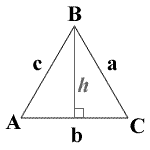## Triangle theorems calculator

 A = B = C = 0
Show Steps

The triangle theorems calculator to find the properties of a triangle given other properties of a triangle.a = side a, b = side b, c = side c

A = angle A, B = angle B, C = angle C, K = area, P = perimeter

Sometimes, you could see AAA ASA SAS AAS SSS, They meanings:

AAA = angle, angle, angle
ASA = angle, side, angle
SAS = side, angle, side
AAS = angle, angle, side
SSS = side, side, side

For exmaple, to SAS: Given two sides and the angle between those sides find the remaining sides and angles.c = 3, B = 60 degree, a = 6:

To calculate A, use the following formula: arccos(b2 + c2 - a2)/2bc. When you substitute a, b, and c for their values, you get arccos((602 + 62 - 32) / (2*60*6)), which equals 90.00005°.

Since a triangle's angles must add up to equal 180°, C must make the sum of all three angles equal 180.
180 - 3 = 89.99995
89.99995 - 60 = 29.99995
The third angle's measurement is 29.99995°.

Calculating K:
To calculate K, you must first find s. To find s, use the following formula: s = (a+b+c)/2.
(a+b+c) = 14.19616 and 14.19616/2 = 7.09808, so s = 7.09808.
Now that you've found s, you may begin calculating the area. The formula for calculating the area of a triangle is
K = √(s*(s-a)(s-b)(s-c)).
First, plug your side length values into the formula: K = √(7.09808*(7.09808-6)(7.09808-5.19615)(7.09808-3).
Next calculate all the calculate all the values in the parentheses:
K = √(7.09808*1.09808*1.90193*409808).
Afterwards, multiple all the numbers together: √(60.7505).
Finally, find the square root of the result for your answer: K = 7.79426 m2.

Calculating P:
To calculate P, add all of the sides together: 6 + 5.19615 + 3 = 14.19615 m.
P = 14.19615 m.

Thinkcalculator.com provides you helpful and handy calculator resources.